# When Regressions Attack

This lesson is all about what can go wrong in linear regression. Here's an outline of the ways things can go wrong.

• data isn't linear
• extreme outliers
• multicolinnearity
• conditioning on a collider
• counfounder bias
• non-normal residuals

There's also a problem known as "autocorrelation" which mainly appears in time series data (i.e., when one tries to run a regression on something that changes over time, like stock market prices). Time series analysis is a fairly advanced topic that is beyond the scope of this course, but you should have alarm bells ringing if anyone tries to do ordinary linear regression on data that spans time like that.

Often, this material is taught in terms of "assumptions of regression." We say that linear regression assumes certain things about your data/the world that your data represent. This is kind of indirect language, however. When stats people talk about "assumptions" what they really mean are "things that have to be true or the regression gets the wrong answer." So we'll just directly talk about the wrong answer and how it can be detected or avoided.

Another way to think about this is as a core part (along with the discussion of p values and power from last week) of your developing a BS detector for statistical claims, offered by scientific studies, expert witnesses, or otherwise. One way of spewing statistical BS is to report the results of a regression which fails these "assumptions" --- which doesn't actually give you real information. So in order to keep from being taken in by the BS, you need to know how regressions can go wrong.

We will also cover some of the major diagnostic methods for identifying these problems, however, this will be far from comprehensive. Statsmodels has a list of the various diagnostics that are built in; it's never wrong to google some of those to learn more.

## Linear Regression: read the first word again.¶

In a lot of ways, the fundamental technical flaw of bad linear regressions is to use it to attempt to represent nonlinear relationships. Remember that what a linear regression does is draws a line (in two dimensions, a plane, or a hyperplane (wooooo) or such in more dimensions) that best allows us to predict the dependent variable from the independent variables. But what if the relationship isn't linear?

There's a very famous set of data visualizations, called Anscombe's quartet, that illustrates this. It's so important that they actually built it into seaborn, so we can just directly run an example plot from their own documentation and take a look:

In :
import seaborn as sns
import pandas as pd
import numpy as np
import matplotlib.pyplot as plt
%matplotlib inline
import statsmodels.formula.api as sm

# Show the results of a linear regression within each dataset
sns.lmplot(x="x", y="y", col="dataset", hue="dataset", data=df,
col_wrap=2, ci=None, palette="muted", height=4,
scatter_kws={"s": 50, "alpha": 1})

Out:
<seaborn.axisgrid.FacetGrid at 0x100de2c18>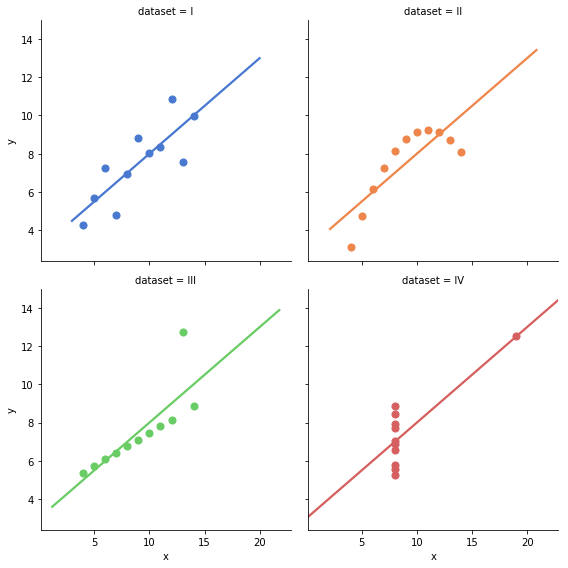Anscombe's quartet is really deep (check out the wikipedia page for details). What you're looking at is four distinct datasets that have identical means and variances in both x and y, identical correlation coefficients between x and y, and identical linear regression coefficients with identical r-squareds. (!!!!!)

We're going to keep coming back to this, but for now I just want you to focus on the top row, and compare the top left with the top right.

The top left is a great linear regression dataset. The relationship looks very linear, in the sense that when x increases, y increases, and it looks like by about the same amount, give or take, each time.

The top right is a terrible linear regression dataset. You can visibly see that the line that OLS is trying to fit here doesn't even remotely match the shape of the data. What this means is that you'll get regression coefficients out of this, but they won't mean very much at all, because there isn't actually relationship between x and y about which we can say "when x changes by some amount, y changes by some other amount"; or, at least, if there is such a range it doesn't exist over the entire range of x.

Sometimes, data transformations can be used to make nonlinear data fit a linear form. The log transformation we looked at a few weeks ago, for example, is pretty good at suppressing some kinds of nonlinearity, though at the cost of making our regression coefficients a bit harder to figure out.

Here, we might try a polynomial transformation, that is, instead of fitting the regression on x, maybe fit it on x squared, or x cubed. That can allow the regression line to stop being, well, a line, and turn into a curve, which can more closely fit the data. Indeed, this will turn out to be a pretty good match for this example.

In :
# first let's carve out all the quartets into separate datasets
quartet1 = df[df["dataset"] == "I"]
quartet2 = df[df["dataset"] == "II"]
quartet3 = df[df["dataset"] == "III"]
quartet4 = df[df["dataset"] == "IV"]

In :
quartet2

Out:
dataset x y
11 II 10.0 9.14
12 II 8.0 8.14
13 II 13.0 8.74
14 II 9.0 8.77
15 II 11.0 9.26
16 II 14.0 8.10
17 II 6.0 6.13
18 II 4.0 3.10
19 II 12.0 9.13
20 II 7.0 7.26
21 II 5.0 4.74
In :
sns.regplot(x="x", y="y", data=quartet2, order=2)

Out:
<matplotlib.axes._subplots.AxesSubplot at 0x10a631668>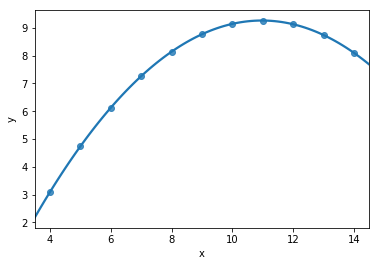In that code, the order=2 parameter told seaborn to, behind the scenes, fit a polynomial regression with order 2 (that is, with a squared independent variable---order 3 would be a cubed independent variable) to the data. So this would more accurately reflect our data, but the price (as with all such transformations) would, yet again, be that the coefficients would be harder to interpret. (When x-squared increases by A, y increases by B... huh?)

There's also another price to using higher-order regressions. Very high-order polynomials are likely to "overfit." What's overfitting? Look at the first dataset in anscombe's quartet again. What would happen if instead of drawing a straight diagonal line, you drew a line connecting every single point?

(discussion of overfitting and advantage of parameters in terms of importing assumptions about general form of data rather than just reflecting the data you have, generalization.)

In :
quartet1 = df[df["dataset"] == "I"]
sns.regplot(x="x", y="y", data=quartet1, order=2)

Out:
<matplotlib.axes._subplots.AxesSubplot at 0x101719828>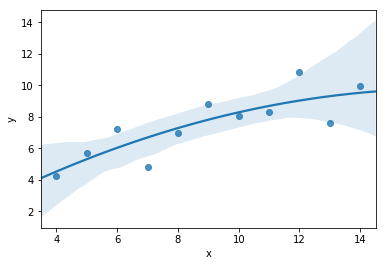What the high-order polynomials are basically doing here is effectively fitting a line to that runs through more of the points. But every dataset has error in it, whether it's measurement error or error (variance, really) rooted in the fact that you have a sample.

By fitting a high degree polynomial, effectively you force the regression to take account of more of the variation between data points. This is good when that variation is real variation, i.e., reflects the underlying process that generates the data (the thing the scientist is trying to study). It's bad when the variation is just noise, because noise in your dataset doesn't tell you anything about the real underlying truth in the population. So it's a bad idea to fit the noise.

What this suggests is that a polynomial regression would be a good idea for #2, because it really looks like there's a kind of polynomial form to the data (a curve). But it would be a bad idea for #1, because a straight line is probably an adequate model for the data.

### Extreme Outliers¶

Go back to Anscombe's quartet at the top of this lesson. Look at the lower two rows. Both are examples of how extreme outliers can distort regression results. The problem is that they'll have large squared errors, and so they'll exercise disproportionate influence on the coefficients you ultimately get.

Outliers are kind of similar to nonlinearity, in the sense that often they will be points radically off from a line that does a good job of fitting the other data. But they need not always represent a nonlinearity---there might be an outlier that lies on the same line as the rest of the data, but just has more extreme values on all dimensions.

Outliers are sometimes also called "influential points."

There are a variety of strategies to deal with outliers. Sometimes, there's good theoretical reason to think they're just measurement error and should be removed. (Remember the people in the Shonubi dataset who were supposedly caught carrying 2000 grams of heroin in a single trip? Uh, no. Someone did a typo that added an extra digit.) Generally, however, that's a bad idea.

In this class, we won't address the alternative strategies (techniques like robust regression) used to accommodate outliers. Detecting them is worthwhile though, and sometimes a bit difficult in multivariate context. (In single-variable regression you can just use a scatterplot.) Statsmodels provides an influence plot which can be used to try to pick out individual points.

In :
from statsmodels.graphics.regressionplots import influence_plot
results = sm.ols(formula='y ~ x', data=quartet3).fit()

influence_plot(results)

Out: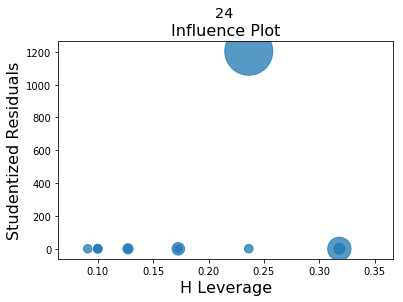In :
results_better = sm.ols(formula='y ~ x', data=quartet1).fit()

influence_plot(results_better)

Out: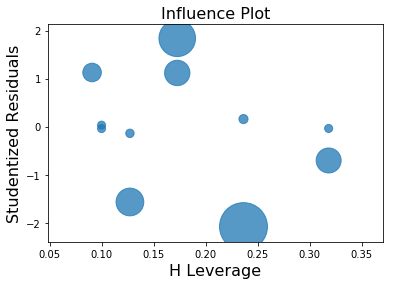## Heteroskedasticity: Scary Word, Simple Idea¶

One problem that can come from nonlinear relationships in your data (and other causes) is known as heteroskedasticity. (Don't ever ask me to try to spell that.) The basic idea here is different residuals along different parts of the range of the data.

There's a classic way to see this, which comes from a scatterplot of the residuals vs fitted values. You'll often see a kind of fan shape in these plots, where as fitted values grow (or shrink) the residuals increase.

Here's an example. We'll pull down my book dataset again just for an easy dataset with more variables in it.

In :
rol = pd.read_csv("http://rulelaw.net/downloads/rol-scores.csv")
rol.columns = [x.lower().strip() for x in rol.columns]

Out:
state pop. in millions for 2012 rolscore elec_pros pol_plur free_expr assoc_org per_auto 2012gdp hprop hfisc hbiz hlab htra hinv
0 Albania 3.2 42.60 8 10 13 8 9 1.264810e+10 30 92.6 81.0 49.0 79.8 65
1 Argentina 41.1 51.94 11 15 14 11 13 4.755020e+11 15 64.3 60.1 47.4 67.6 40
2 Australia 22.7 73.28 12 15 16 12 15 1.532410e+12 90 66.4 95.5 83.5 86.2 80
3 Austria 8.4 73.15 12 15 16 12 15 3.947080e+11 90 51.1 73.6 80.4 86.8 85
4 Bangladesh 154.7 31.57 9 11 9 8 9 1.163550e+11 20 72.7 68.0 51.9 54.0 55

Annoyingly, there isn't a library method to do a fitted vs residual plot, but we can do it ourselves very easily.

In :
def res_vs_fitted(x, y, data):
formula = '{} ~ {}'.format(y, x)
res = sm.ols(formula=formula, data=data).fit()
return plt.scatter(res.fittedvalues, res.resid)


Ok, let's start by looking at a plot that lacks heteroskedasticity.

In :
res_vs_fitted(x="elec_pros", y="pol_plur", data=rol)

Out:
<matplotlib.collections.PathCollection at 0x1023934a8>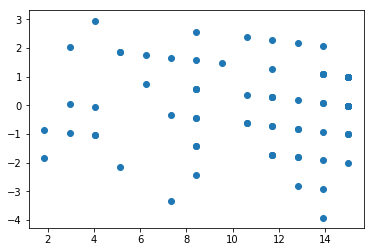Note how there's no definiable pattern in the plot of residuals on fits. That's what you want. It's a cloud. An amorphous, lovely, cloud.

But now, let's screw up the data. we'll define a function that makes things noisier as the value increases.

In :
from copy import deepcopy
def make_heteroskedasticity(x, y, data):
# just making sure I don't accidentally manipulate the underlying data, which
# is always a danger with pandas, and too lazy to figure out how to ensure that
# inside the dataframe.
breakx = deepcopy(list(data[x]))
breaky = deepcopy(list(data[y]))
stdy = np.std(breaky)
tw5 = np.percentile(breakx, 25)
median = np.percentile(breakx, 50)
sv5 = np.percentile(breakx, 75)
# now we're going to add more noise to y values corresponding to higher x values.
for idx, item in enumerate(breakx):
if (item > tw5) and (item < median):
breaky[idx] += np.random.normal(loc=0.0, scale=stdy)
elif (item > median) and (item < sv5):
breaky[idx] += np.random.normal(loc=0.0, scale=2 * stdy)
elif item > sv5:
breaky[idx] += np.random.normal(loc=0.0, scale=3 * stdy)
return np.array(breaky)

rol['broken_pol_plur'] = make_heteroskedasticity("elec_pros", "pol_plur", rol)

Out:
broken_pol_plur pol_plur elec_pros
0 4.651249 10 8
1 5.472289 15 11
2 15.000000 15 12
3 15.000000 15 12
4 11.615242 11 9

That should be nice and messed up. Let's see!

In :
res_vs_fitted(x="elec_pros", y="broken_pol_plur", data=rol)

Out:
<matplotlib.collections.PathCollection at 0x10ac316a0>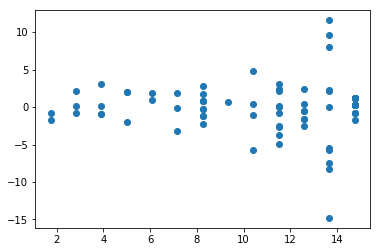Ok, so this was a contrived example, but it works: look how the residuals get more dispersed as we get to higher fitted values. That's the classic fan shape, and it reveals bad heteroskedasticity. (As well it ought to, since I imposed it on the data by force.)

The consequence of heteroskedasticity is that our p values will be too low---essentially, OLS will be underestimating the amount of variance in the data, and thus concluding that coefficients are more signifiant than they really are. Practically speaking, what that means is that finding heteroskedasticity is a great way to bust up a supposed significant result.

Incidentally, a residual vs fitted plot is also a great way to detect nonlinear relationships. For example!

In :
rol["gdppc"] = rol["2012gdp"] / rol["pop. in millions for 2012"]

In :
res_vs_fitted(x="rolscore", y="gdppc", data=rol)

Out:
<matplotlib.collections.PathCollection at 0x10ad50470>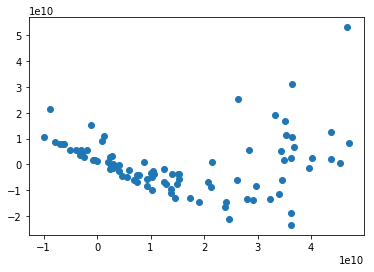## Multicollinearity¶

This is another fancy name for a simple problem: when some of the independent (right-side) variables in a regression model are highly correlated with one another. This is a problem, essentially, because it means that it's meaningless to talk about the independent effect of them on your regression. Put differently, if one variable is highly correlated with another so that they're basically measuring the same thing, then when you include them both in your model, they're effectively controlling one another away, leading to nonsensical results.

An easy way to make this mistake is just to put a variable and some linear transformation of that variable into the model---for example, y ~ xa + xb + xc where xc = 3 * xa --- in effect, you're saying that the coefficient on x should be how the value of y changes when xa changes, holding 3 * xa constant, which is obviously nonsensical. But it's possible to fall into multicollinearity problems in less obvious ways.

In :
rol["elec_b"] = rol["elec_pros"] * 5
dumb_formula = "rolscore ~ elec_pros + pol_plur + elec_b"
multicollinear_mess = sm.ols(formula=dumb_formula, data=rol).fit()
print(multicollinear_mess.summary())

                            OLS Regression Results
==============================================================================
Dep. Variable:               rolscore   R-squared:                       0.467
Method:                 Least Squares   F-statistic:                     38.61
Date:                Sat, 30 Mar 2019   Prob (F-statistic):           9.18e-13
Time:                        16:01:41   Log-Likelihood:                -351.15
No. Observations:                  91   AIC:                             708.3
Df Residuals:                      88   BIC:                             715.8
Df Model:                           2
Covariance Type:            nonrobust
==============================================================================
coef    std err          t      P>|t|      [0.025      0.975]
------------------------------------------------------------------------------
Intercept     21.9994      3.607      6.098      0.000      14.830      29.168
elec_pros     -0.0666      0.040     -1.678      0.097      -0.145       0.012
pol_plur       3.9319      0.888      4.426      0.000       2.166       5.697
elec_b        -0.3328      0.198     -1.678      0.097      -0.727       0.061
==============================================================================
Omnibus:                        6.363   Durbin-Watson:                   1.901
Prob(Omnibus):                  0.042   Jarque-Bera (JB):                2.912
Skew:                           0.131   Prob(JB):                        0.233
Kurtosis:                       2.164   Cond. No.                     7.86e+16
==============================================================================

Warnings:
 Standard Errors assume that the covariance matrix of the errors is correctly specified.
 The smallest eigenvalue is 3.63e-29. This might indicate that there are
strong multicollinearity problems or that the design matrix is singular.


Note that statsmodels warns you about the risk of a multicollinearity problem (you can't rely on this to always happen, but sometimes it will do so).

Let's also compare this to a non-idiotically-specified model.

In :
nondumb_formula = "rolscore ~ elec_pros + pol_plur"
lessdumb = sm.ols(formula=nondumb_formula, data=rol).fit()
print(lessdumb.summary())

                            OLS Regression Results
==============================================================================
Dep. Variable:               rolscore   R-squared:                       0.467
Method:                 Least Squares   F-statistic:                     38.61
Date:                Sat, 30 Mar 2019   Prob (F-statistic):           9.18e-13
Time:                        16:01:41   Log-Likelihood:                -351.15
No. Observations:                  91   AIC:                             708.3
Df Residuals:                      88   BIC:                             715.8
Df Model:                           2
Covariance Type:            nonrobust
==============================================================================
coef    std err          t      P>|t|      [0.025      0.975]
------------------------------------------------------------------------------
Intercept     21.9994      3.607      6.098      0.000      14.830      29.168
elec_pros     -1.7308      1.032     -1.678      0.097      -3.781       0.319
pol_plur       3.9319      0.888      4.426      0.000       2.166       5.697
==============================================================================
Omnibus:                        6.363   Durbin-Watson:                   1.901
Prob(Omnibus):                  0.042   Jarque-Bera (JB):                2.912
Skew:                           0.131   Prob(JB):                        0.233
Kurtosis:                       2.164   Cond. No.                         45.8
==============================================================================

Warnings:
 Standard Errors assume that the covariance matrix of the errors is correctly specified.


Note how the coefficient on the elec_pros variable is different. Also notice the "Cond. No." at the bottom of the summary results. This is the "condition number," a common indicator of multicollinearity; in the previous regression it was HUGE (something with 16 zeroes after it). Now it's 45. Actually, a standard rule of thumb that people use is that any condition number over 30 gives you reason to worry about multicollinearty, so we might not even be out of the weeds here.

This actually shouldn't be surprising, given the nature of the underlying variables--- "electoral process" and "political pluralism" in this dataset are both indicators of a country's underlying democratic freedoms, so we'd expect them to be fairly highly correlated. Indeed, there are multicollinearity problems in most regressions we might try for the rule of law dataset, because the underlying variables just happen to be closely related to one another.

That being said, the statsmodels condition number functionality appears to be a little bit noisy---it reports high condition numbers sometimes even in single-variable regression, so I wouldn't trust it too much. So for present purposes we'll put this in the "not going to deal with it further; google it and use R if it comes up in real life" box.

Sometimes, there can be worries about multicollinearity when including interaction terms in a model. Interaction terms are multiplying variables together, for example, y ~ xa + xb + xc + xa * xb. This is a common technique to capture situations where the theory suggests that the impact of one independent variable on the outcome will depend on the value of another variable. For example, suppose you think that women are discriminated against more when they have more education, because men are threatened by smart women---such that education might be a disadvantage for women but an advantage for men. One way to capture this would be in an interaction term between years of education and a binary (indicator) variable for gender.

To reduce the risk of multicollinearity with interaction terms, some people advise either leaving off the un-interacted variables (i.e., leave off the variables for gender and for education, leaving just the interaction), others suggest mean-centering the variables (subtracting the mean of the variable from each value of it). These are also sometimes suggested as a way to handle multicollinearity risks from quadratic terms (like squaring an independent variable). But the mean-centering thing is debatable, and dropping other terms might not capture the theory underlying the model. Many stats folks just recommend gathering more data covering more extreme values of the underlying variables, so that the interaction/quadratic terms will vary more widely from their underlying components, hopefully meaning less multicollinearity.

Also, as long as you don't have extreme multicollinearity (i.e., measuring the same thing multiple ways), having somewhat correlated variables won't necessarily make a regression do anything truly terrible... the mostly common problem is that it will inflate standard errors, and hence make it much harder to detect statistically significant effects---because, as noted, effectively you'll have a variable controlling itself away.

### Conditioning on a Collider¶

A sort of vaguely related idea to multicollinearity (in the sense that we're worried about controlling away the effects we're trying to measure) is conditioning on a collider (also known as "collider bias").

What's a collider? It's something that is influenced (in a causal sense) both by the dependent variable and by an independent variable. For example, suppose you're doing a study of whether race influences the number of times a person reports having ever been stoped by the police. Degree of reported trust in the police would probably be a collider there, because both being a member of a minoritized race and being stopped by the cops would be likely to increase the chance of distrusting the police.

What's conditioning? It's, loosely speaking (being a bit hand-wavey in order to avoid reintroducing technical probability stuff), making your estimation depend on it or changing your estimation procedure because of it. This includes selecting cases depending (intentionally or unintentionally) on their value with respect to the collider (this is a common issue in experimental studies that use convenience samples, like college students forced to take psych studies in a class, where being included in the sample population might be a collider), and also includes putting the collider on the right side of your regression model. In the example above, either putting trust in your regression or studying a sample where inclusion depends on whether they say they distrust the cops would be conditioning on a collider.

A related problem is conditioning on a post-treatment variable in an experimental context, that is, conditioning on a variable that might be influenced by the treatment, for example, by dropping subjects who don't comply with the instructions in the experiment. Also a bad idea (known as "post-treatment bias").

Both of these are bad and can totally mess over results.

## Counfounder Bias¶

By contrast, omitted variable bias is a general term for variables that you ought to include because not doing so breaks the results of your regression---because they also might cause your dependent variable, and if they're associated with the independent variable in the wrong kinds of ways, leaving them off can distort the results of our regression. Remember our Simpson's Paradox example from a couple of weeks ago!

The most straightforward kind of omitted variable bias is confounder bias. A confounder is a variable that exercises a causal influence on both the dependent variable and an independent variable. For example, suppose you're studying whether taking extended periods of time off work affects satisfaction with management using a regression. It's fairly likely that gender affects both of those things---the first because of pregnancy, and the second because of sexual harassment and other kinds of workplace misconduct directed against women. So you should include it on the right side of your regression.

Further reading: Catalog of Bias: Confounding

## Non-Normal Residuals¶

P-values and such only work in OLS regression if the residuals are normally distributed around zero. A quick and easy way to check for this is to do what's known as a q-q plot or (slightly different but serves the same purpose, a probability plot can serve), plotting the residuals of your model against a normal. For example:

In :
import statsmodels.api as sm_api # NOT the formula API that we gave to the name sm
basic_regression = sm.ols(formula="rolscore ~ elec_pros + per_auto + hprop", data=rol).fit()
residuals = basic_regression.resid
sm_api.qqplot(residuals)

Out: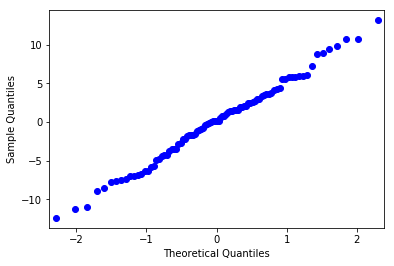This looks pretty good---like a nice straight line. By contrast, if we do a kind of garbage regression (just contrived using some of my artificial terrible variables from before, because I expect that it will break all the things):

In :
bad_regression = sm.ols(formula="assoc_org ~ gdppc + broken_pol_plur + elec_pros + elec_b", data=rol).fit()

Out: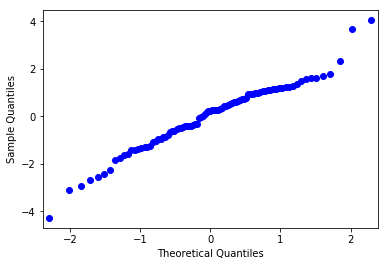That looks a little worse, especially on the extremes. Further reading on this plot and a number of other useful diagnostic plots: this explainer from the University of Virginia describes them using the R language.

## Some built-in diagnostics¶

Let's look at some of the summary data that statsmodels gives us now. Here's that summary again:

In :
print(basic_regression.summary())

                            OLS Regression Results
==============================================================================
Dep. Variable:               rolscore   R-squared:                       0.885
Method:                 Least Squares   F-statistic:                     223.6
Date:                Sat, 30 Mar 2019   Prob (F-statistic):           9.06e-41
Time:                        16:01:42   Log-Likelihood:                -281.32
No. Observations:                  91   AIC:                             570.6
Df Residuals:                      87   BIC:                             580.7
Df Model:                           3
Covariance Type:            nonrobust
==============================================================================
coef    std err          t      P>|t|      [0.025      0.975]
------------------------------------------------------------------------------
Intercept     11.6512      2.050      5.685      0.000       7.577      15.725
elec_pros     -0.5598      0.265     -2.114      0.037      -1.086      -0.033
per_auto       2.9828      0.415      7.189      0.000       2.158       3.808
hprop          0.2797      0.040      7.000      0.000       0.200       0.359
==============================================================================
Omnibus:                        0.185   Durbin-Watson:                   2.039
Prob(Omnibus):                  0.912   Jarque-Bera (JB):                0.372
Skew:                          -0.001   Prob(JB):                        0.830
Kurtosis:                       2.687   Cond. No.                         201.
==============================================================================

Warnings:
 Standard Errors assume that the covariance matrix of the errors is correctly specified.


I want to add several caveats to this discussion of the statsmodels results output.

Caveat 1. Statsmodels has, frankly, terrible documentation. And a number of the tests in the third row could be applied to a number of different things (i.e., to the distribution of the residuals from our model, as opposed to, say, the dependent variable or something), and the document doesn't specifically say what statsmodels applies them to to generate the summary output. I'm going to assume that they are being applied to the things they're supposed to be applied to, because the people who wrote statsmodels aren't stupid. This is almost certainly a safe assumption, but I really don't like making any assumptions, so, in a critical context, I'd probably want to look up the statsmodels functions for a particular test and apply them directly to the residuals myself.

Honestly, though, for regressions I'd probably just use a different language---R has much more fleshed-out documentation as well as the advantage of being used by a truly large number of stats people, so that the toolkit for basic models like regression has been combed over by countless experts. If I were teaching a full-scale regression analysis class (as opposed to just touching on it in the context of lots of other things where a real general-purpose programming language is more useful than a specialized stats language) I'd teach it in R. And if you find yourself doing regressions seriously, go learn R and do them in there.

Caveat 2. No single diagnostic number or even collection of diagnostic numbers will tell you whether a regression is usefully specified or not. These are cues to further investigate, and to adjust (like a good Bayesian) your ultimate belief in the propositions that the model is supposed to support.

Caveat 3. We're not digging into this in a lot of detail here. And we probably won't have time to discuss this stuff in class. For practical lawyering purposes, the main point of seeing this kind of material is just to have an extra couple of questions to ask, or possibly triggers of things to worry about, for example, when you see an expert report.

With those caveats in mind:

The omnibus test is a hypothesis test with the null hypothesis that a distribution is normal. Assuming statsmodels is sensible here, this hypothesis test is being applied to the residuals, so the important thing is the prob(omnibus) entry, and a low p-value suggests that we should probably worry about having non-normal residuals. The Jarque-Bera test is another test for normality.

The Durbin-Watson statistic is a measure of autocorrelation. As I said, this is mostly important in time series data, which comes up most commonly in the context of financial modeling---I understand from the folks who do this work that the range of this statistic is 0-4, and that the closer to 2, the less likely that there's a worry about autocorrelation; generally 1.5-2.5 is ok.

Skew and Kurtosis are measures of how far off a distribution is from normal---skew is about whether it's not symmetrical (like more data on the left or right), kurtosis is about how spiky it is. A normal distribution should have skew around zero; and a standard normal will have kurtosis of 3. Here's a good read from NIST on skew and kurtosis. You'll hear different things about acceptable ranges of each.

We've already talked about the condition number---and about how the example data is just full of All the Multicollinearity.

These aren't the only regression diagnostic tests statsmodels offers---check out their documentation for more. But this will do as a summary for now.